Module 24 - Answers Lesson 1 Answer 1 24.1.1   1 + x 1 + x + x2 1 + x + x2 + x3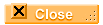Answer 2 24.1.2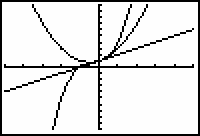The graphs appear to coincide when x is between -0.5 and 0.5.Answer 3 24.1.3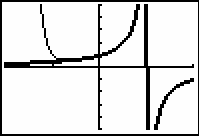Answer 4 24.1.4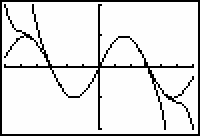The partial sums appear to converge to y = sin x.Answer 5 24.1.5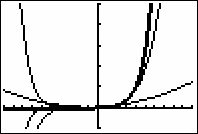[-10,10,1] x [-50,250,50] As more terms are added, the interval where each partial sum matches ex widens.Lesson 2 Answer 1 24.2.1 f(-0.5)0.60653 p(-0.5) = 0.625 f(0.5)1.6487 p(0.5) = 1.625 In each case, the values of the function and the approximating polynomials are close.Answer 2 24.2.2   Letting f(x) = ln(1 + x), the table below lists the coefficients of the fifth-order Maclaurin polynomial.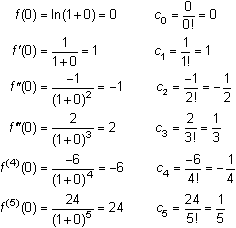The fifth-order Maclaurin polynomial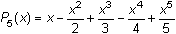approximates f(x) = ln(1 + x) for values of x close to 0.Answer 3 24.2.3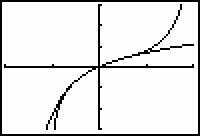[-2, 2, 1] x [-3, 3, 1]Lesson 3 Answer 1 24.3.1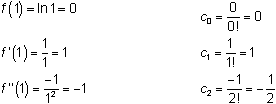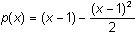is the second-order Taylor polynomial for ln x centered at 1.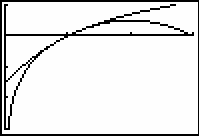[0, 3, 1] x [-3, 1, 1]Answer 2 24.3.2 1 - (x - 1) + (x - 1)2 is the second-order Taylor polynomial for 1/x centered at 1.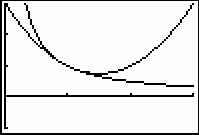[0, 3, 1] x [-1, 3, 1]Self Test Answer 1 The fifth-order Maclaurin polynomial for tan-1(x) is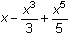. Answer 2 The interval of convergence appears to be (-1,1).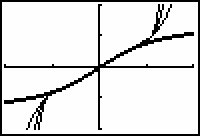[-2, 2, 1] x [-2, 2, 1] Answer 3 The second-order Taylor polynomial centered at 2 is for ex is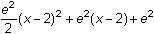. Answer 4 The fourth-order Maclaurin polynomial for ex2 is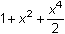. Answer 5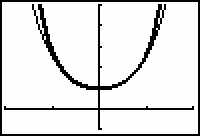[-2, 2, 1] x [-1, 5, 1]©Copyright 2007 All rights reserved. | Trademarks | Privacy Policy | Link Policy